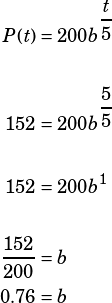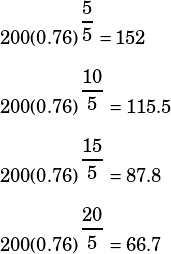Hey Mike, could you please help me with practise test 8, section 4 question 38? If you simply plug in P as 152, it’s easy to get the answer. However, im not sure what “if P approximates the values in the table to within 10 micrograms per milliliter” exactly means? How does that statement help me to solve the question or change the numbers I’m inputting as P?

Thank you so much!

I think you’ve got the key here already: recognizing that P is supposed to approximate all the values in the graph/table, so start with the easy ones! The table tells you that from minute 0 to minute 5, the penicillin concentration goes from 200 to 152 micrograms per milliliter. As you said, plug those values into the formula:Now use your calculator to quickly test whether settinggives you values within 10 micrograms per milliliter to each of the values in the table.Indeed, each of those numbers is well within 10micrograms per milliliter of the values in the table. Now all we need to do is remember to round to nearest tenth! 0.76 rounded to the nearest tenth is 0.8, which is the answer.

Note: If you rounded to the nearest tenth first, you’d actually still be OK:That’s a little worse, but each of those values is still within 10 of the values in the table (OK, so 128 is exactly 10 from 118). Trying 0.7 or 0.9 would give you additional comfort that 0.8 is the right answer.